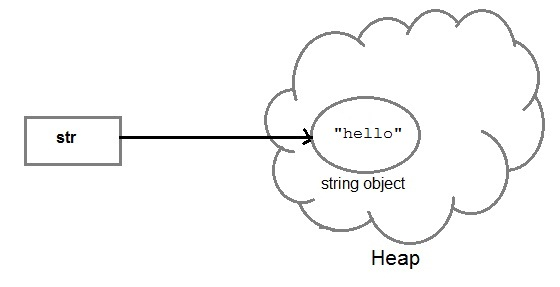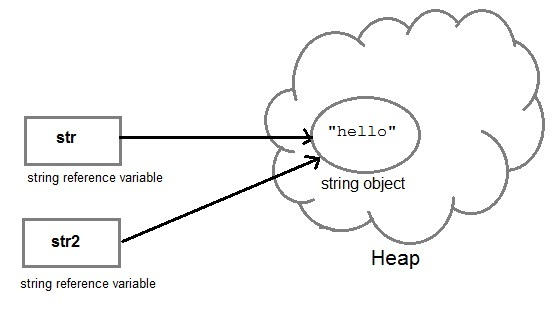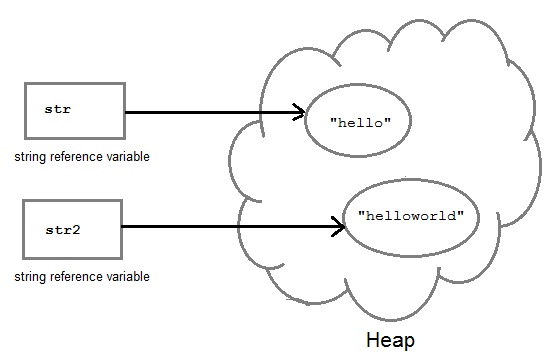Core JAVA

### Introduction to String Handling

String is probably the most commonly used class in java library. String class is encapsulated under `java.lang` package. In java, every string that you create is actually an object of type String. One important thing to notice about string object is that string objects are immutable that means once a string object is created it cannot be altered.

#### What is an Immutable object?

An object whose state cannot be changed after it is created is known as an Immutable object. String, Integer, Byte, Short, Float, Double and all other wrapper class's objects are immutable.

#### Creating an Immutable class

```public final class MyString
{
final String str;
MyString(String s)
{
this.str = s;
}
public String get()
{
return str;
}
}
```
In this example MyString is an immutable class. MyString's state cannot be changed once it is created.

#### Creating a String object

String can be created in number of ways, here are a few ways of creating string object.

#### 1) Using a String literal

String literal is a simple string enclosed in double quotes `" "`. A string literal is treated as a String object.

```String str1 = "Hello";
```

#### 2) Using another String object

```String str2 = new String(str1);
```

#### 3) Using new Keyword

```String str3 = new String("Java");
```

#### 4) Using + operator (Concatenation)

```String str4 = str1 + str2;
or,
String str5 = "hello"+"Java";
```

Each time you create a String literal, the JVM checks the string pool first. If the string literal already exists in the pool, a reference to the pool instance is returned. If string does not exist in the pool, a new string object is created, and is placed in the pool. String objects are stored in a special memory area known as string constant pool inside the heap memory.

#### String object and How they are stored

When we create a new string object using string literal, that string literal is added to the string pool, if it is not present there already.

`String str= "Hello";`And, when we create another object with same string, then a reference of the string literal already present in string pool is returned.

`String str2=str;`But if we change the new string, its reference gets modified.

`str2=str2.concat("world");`#### Concatenating String

There are 2 methods to concatenate two or more string.

1. Using concat() method
2. Using `+` operator

#### 1) Using concat() method

```string s = "Hello";
string str = "Java";
string str2 = s.concat(str);
String str1 = "Hello".concat("Java");    //works with string literals too.
```

#### 2) Using + operator

```string str = "Rahul";
string str1 = "Dravid";
string str2 = str + str1;
string st = "Rahul"+"Dravid";
```

#### String Comparison

String comparison can be done in 3 ways.

1. Using equals() method
2. Using `==` operator
3. By CompareTo() method

#### Using equals() method

equals() method compares two strings for equality. Its general syntax is,

```boolean equals (Object str)
```

It compares the content of the strings. It will return true if string matches, else returns false.

```String s = "Hell";
String s1 = "Hello";
String s2 = "Hello";
s1.equals(s2);    //true
s.equals(s1) ;   //false
```

#### Using == operator

`==` operator compares two object references to check whether they refer to same instance. This also, will return true on successful match.

```String s1 = "Java";
String s2 = "Java";
String s3 = new string ("Java");
test(Sl == s2)     //true
test(s1 == s3)      //false
```

#### By compareTo() method

compareTo() method compares values and returns an int which tells if the string compared is less than, equal to or greater than th other string. Its general syntax is,

```int compareTo(String str)
```

To use this function you must implement the Comparable Interface. compareTo() is the only function in Comparable Interface.

```String s1 = "Abhi";
String s2 = "Viraaj";
String s3 = "Abhi";
s1.compareTo(S2);     //return -1 because s1 < s2
s1.compareTo(S3);     //return 0 because s1 == s3
s2.compareTo(s1);     //return 1 because s2 > s1
```